# Yesterday End of Carbon Nitrogen cycles section Carbon

• Slides: 18Yesterday End of Carbon & Nitrogen cycles section Carbon Cycle quiz Nitrogen Cycle worksheet Today Population slides – definitions and concepts Population video https: //www. youtube. com/watch? v=RBOsqm. BQBQk Density calculation worksheet – groups or indiv?Ecology - Populations Population: A group of individuals of the same species inhabiting the same area. Population characteristics: Population Density Population Size Population Age Structure Population Dispersion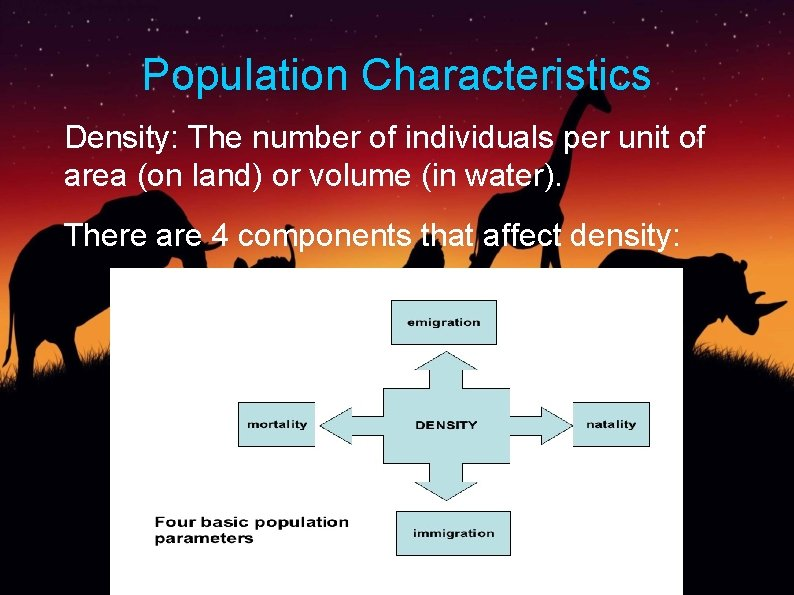Population Characteristics Density: The number of individuals per unit of area (on land) or volume (in water). There are 4 components that affect density: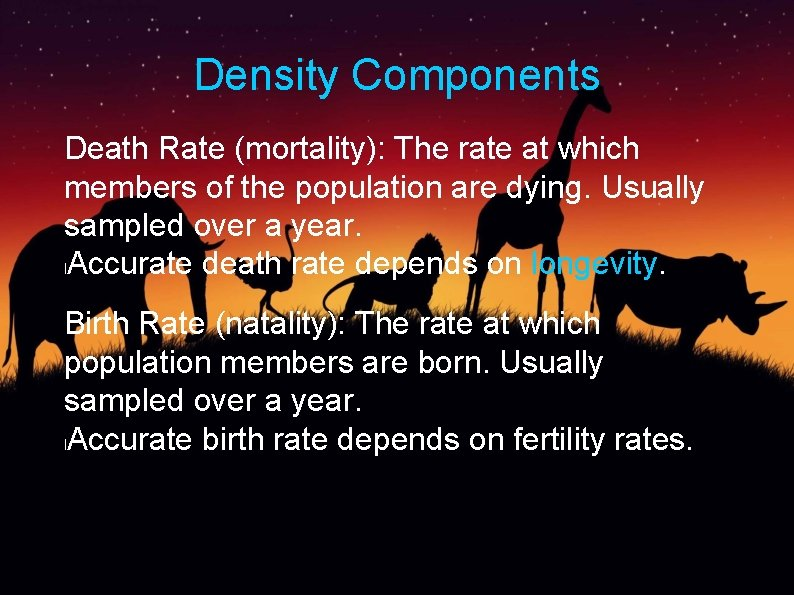Density Components Death Rate (mortality): The rate at which members of the population are dying. Usually sampled over a year. l. Accurate death rate depends on longevity. Birth Rate (natality): The rate at which population members are born. Usually sampled over a year. l. Accurate birth rate depends on fertility rates.Density Components Immigration: The number of organisms moving into an area occupied by the population. Emigration: The number of organisms moving out of the area occupied by the population. Birth Rate & Immigration = Population increases Death Rate & Emigration = Population decreases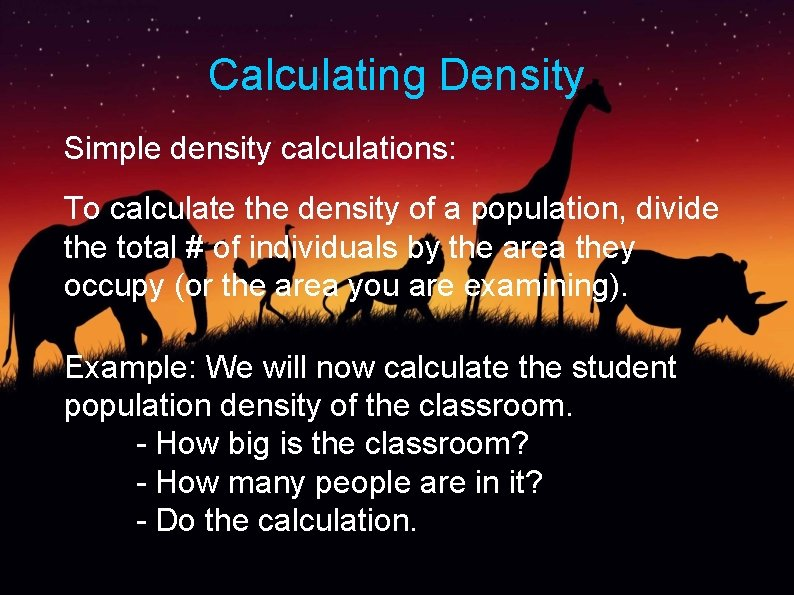Calculating Density Simple density calculations: To calculate the density of a population, divide the total # of individuals by the area they occupy (or the area you are examining). Example: We will now calculate the student population density of the classroom. - How big is the classroom? - How many people are in it? - Do the calculation.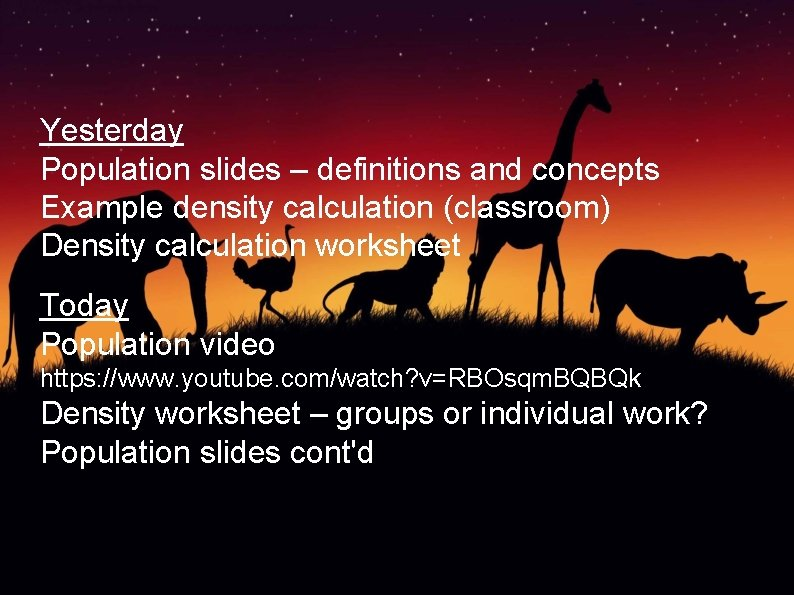Yesterday Population slides – definitions and concepts Example density calculation (classroom) Density calculation worksheet Today Population video https: //www. youtube. com/watch? v=RBOsqm. BQBQk Density worksheet – groups or individual work? Population slides cont'dCalculating Density Why does the density of a population matter? - Absolute density vs Relative density - Helps determine a population's health and growth trends over time. Examples: - Types and numbers of trees in a given area can tell us about the health of a forest. - Crop densities can tell us which type of soil is appropriate for growing which crop.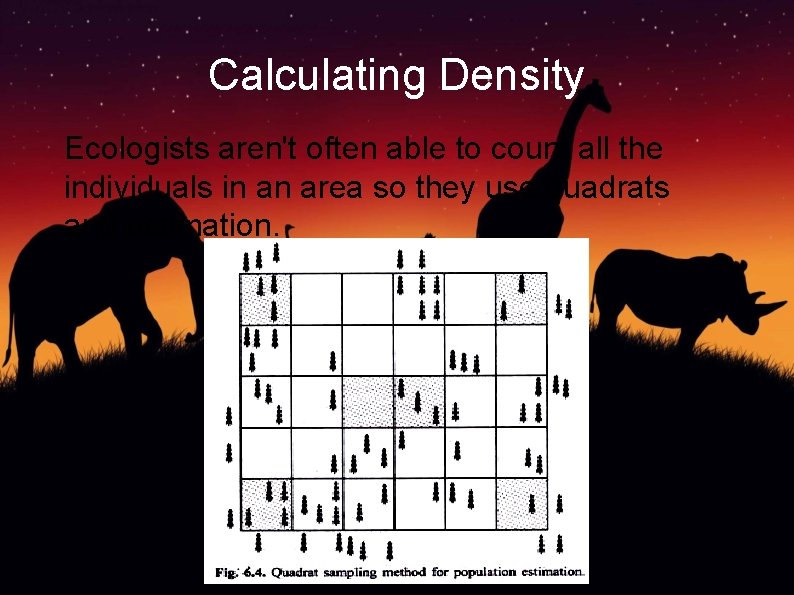Calculating Density Ecologists aren't often able to count all the individuals in an area so they use quadrats and estimation.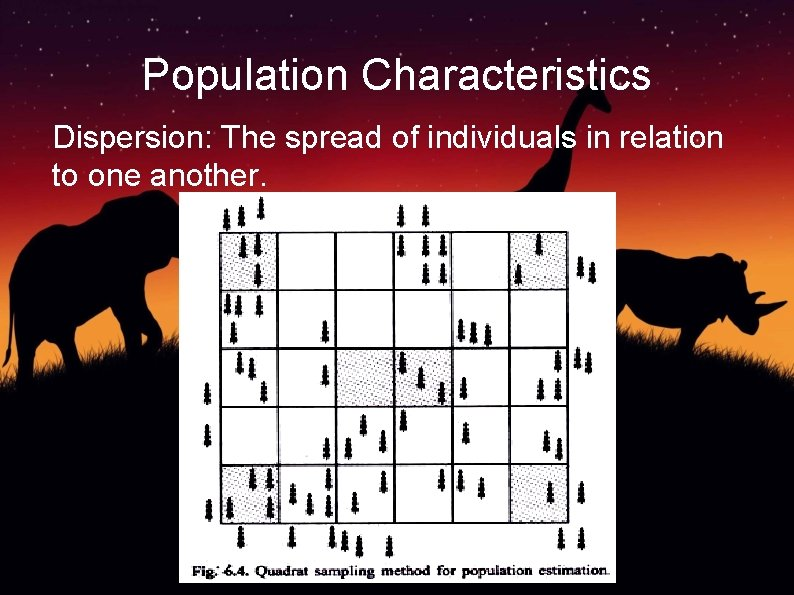Population Characteristics Dispersion: The spread of individuals in relation to one another.Calculating Density Ecologists will also use the Capture & Recapture method. This method requires the capture of a small number of the population. The animals are then tagged / tattooed, and released. Later, more animals are captured and checked for tags. The number of repeat tags gives a good idea of the population density.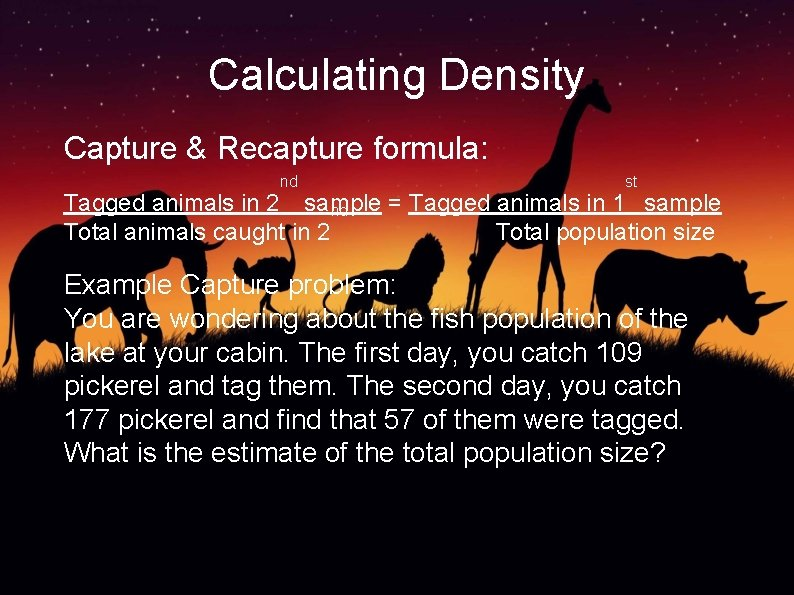Calculating Density Capture & Recapture formula: nd st Tagged animals in 2 sample = Tagged animals in 1 sample nd Total animals caught in 2 Total population size Example Capture problem: You are wondering about the fish population of the lake at your cabin. The first day, you catch 109 pickerel and tag them. The second day, you catch 177 pickerel and find that 57 of them were tagged. What is the estimate of the total population size?Calculating Density Answer: 57 / 177 = 109 / Total pop = (109 x 177) 57 = 338 pickerelPopulation Characteristics Size: The total number of individuals l The size of a population is usually an estimated number. Age distribution: Proportion of different ages of a population. Example: Take the total size of the population and break it into sections by age. How many of each age section can give us a lot of information.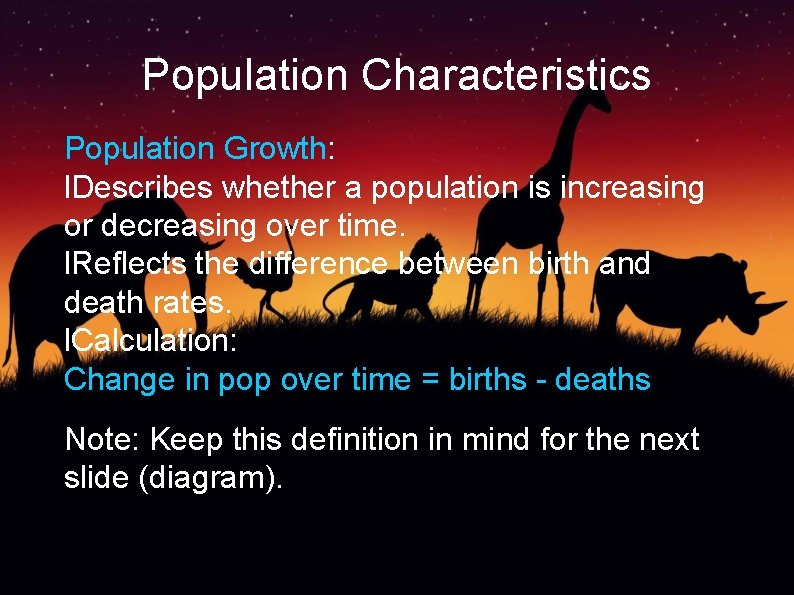Population Characteristics Population Growth: l. Describes whether a population is increasing or decreasing over time. l. Reflects the difference between birth and death rates. l. Calculation: Change in pop over time = births - deaths Note: Keep this definition in mind for the next slide (diagram).Age distribution graphAge distribution graph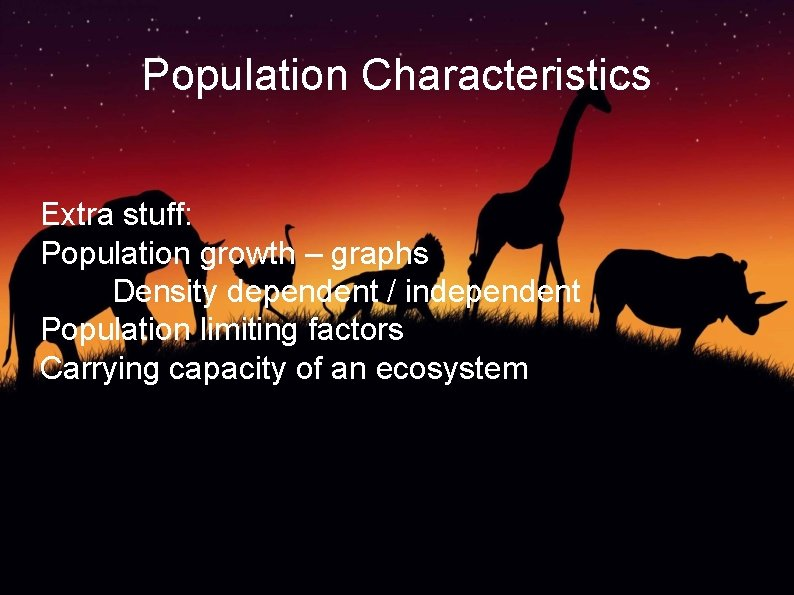Population Characteristics Extra stuff: Population growth – graphs Density dependent / independent Population limiting factors Carrying capacity of an ecosystem